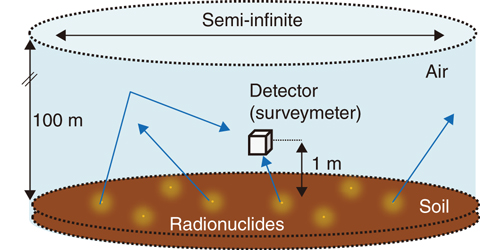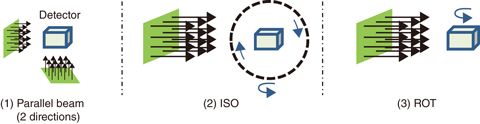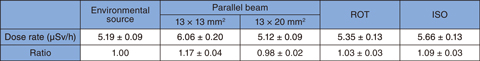# 1-9　Accurate Measurement and Evaluation of Environmental Dose Rates in Air

## －Dose-Rate Evaluation in the Environment using Monte Carlo Simulation－Fig.1-23 The simulation geometry of the environment

The transport of the γ-rays emitted from radionuclides distributed in soil was simulated using the radiation-transport-calculation code PHITS. Dose rates in air were calculated with consideration of the energies and incident directions of γ-rays entering a detector 1 m above the ground.Fig.1-24 Various irradiation conditions of γ-rays

Various irradiation conditions are shown for a CsI(Tl) scintillation detector (13 × 13 × 20 mm3), which is simulated by calculation analysis. The irradiations of the ISO and ROT were performed under the condition where the source was rotated around the detector.

Table 1-1 Comparison of dose rates under various γ-ray-irradiation conditions

Dose rates depend on the irradiation geometry of γ-rays. Ratios of dose rates to those in the environment (environmental dose) are also shown.This Picture(76KB)

Following the TEPCO’s Fukushima Daiichi NPS (1F) accident on March 11, 2011, radiation monitoring has been periodically performed to obtain environmental dose rates in air. Because scintillation-crystal-based detectors such as survey meters are usually used for ensuring safety in nuclear power plants, dose-rate calibration is performed for accurate measurement under conditions where the radiation is incident on a detector from one direction. In contrast, the radiations that were released from radionuclides such as cesium into the environment by the 1F accident and which were widely deposited in soil are incident on the detector from various directions.

Therefore, for the purpose of investigating the necessary calibration condition of detectors for accurate measurement of the doses in the environment, the influence of the incidence direction of radiation upon the dose-rate measurement was analyzed using the particle and heavy-ion transport system (PHITS). First, an environment with radionuclides existing in the soil (Ground source, Fig.1-23) was reproduced on a computer, and the dose rates 1 m above the ground’s surface were calculated. The CsI(Tl)-scintillation detector of crystal size 13 × 13 × 20 mm, was selected for a car-borne survey. Second, the dose rates were calculated under the following irradiation conditions: (1) a uniform parallel-beam condition against each crystal surface (13 × 13 mm2 and 13 × 20 mm2); (2) the condition where a detector is irradiated isotropically from all directions (ISO); and (3) the condition where only the lateral surface of a detector is irradiated laterally (ROT) (Fig.1-24). Furthermore, all calculated results were normalized by the dose rates measured around 1F (Table 1-1 Dose rates for the Ground source).

The results of the calculated dose rates under the irradiation conditions are shown in Table 1-1. The dose rate for the parallel beam irradiation on the 13 × 13 mm2 crystal surface is approximately 17% larger than that for the Ground source. Conversely, the dose rates in the ISO and ROT geometries agree with the dose rate for the Ground source to within 10%; this result indicates that the incident directions of gamma rays in the ISO and ROT geometries are similar to that for the Ground source. Moreover, the parallel-beam irradiation on the 13 × 20 mm2 crystal surface agrees well with the dose rate for the Ground source. It is found from these results that a simple calibration method under unidirectional irradiation can be sufficiently accurate to obtain the dose rates in the environment.

In the future, the dependence of detector configuration on the gamma rays’ incidence direction will be analyzed.

| | | | |